Aims:

1. To enable candidates to acquire knowledge and to develop an understanding of the terms, concepts, symbols, definitions, principles, processes and formulae of Mathematics at the Senior Secondary stage.

2. To develop the ability to apply the knowledge and understanding of Mathematics to unfamiliar situations or to new problems.

3. To develop skills of –

(a) computation.
(b) reading tables, charts, graphs, etc.

4. To develop an appreciation of the role of Mathematics in day-to-day life.

5. To develop an interest in Mathematics.

6. To develop a scientific attitude through the study of Mathematics.

A knowledge of Arithmetic and Pure Geometry is assumed. The parts of Geometry which are of chief importance in other branches of Mathematics are the fundamentals concerning angles, parallels (including lines and planes in space), similar triangles (including the theorem of Pythagoras) the ‘symmetry’ properties of chords and tangents of a circle, and the theorem that a line perpendicular to two non-parallel lines in a plane is perpendicular to every line therein. The examination may include questions with a geometrical content.

As regards the standard of algebraic manipulation, students should be taught:

(i) To check every step before proceeding to the next particularly where minus signs are involved. (ii) To attack simplification piecemeal rather than en block, e.g. never to keep a common factor which can be cancelled. (iii) To observe and act on any special features of algebraic form that may be obviously present.

The standard as regards (iii) is difficult to define; initial practice should be on the easiest cases, ‘trick’ examples should be avoided and it should be kept in mind that (iii) is subsidiary in importance to (i) and (ii) Teachers should be scrupulous in setting a standard of neatness and in avoiding the slovenly habit of omitting brackets or replacing them by dots.

CLASS XI

There will be one paper of three hours duration of 100 marks. The syllabus is divided into three sections A, B and C. Section A is compulsory for all candidates. Candidates will have a choice of attempting questions from either Section B or Section C.

Section A (80 marks) will consist of 9 questions. Candidates will be required to answer Question 1(compulsory) and five out of the rest of the eight questions.

Section B / C (20 marks) Candidates will be required to answer two questions out of three from either Section B or Section C.

SECTION A

1. Mathematical Reasoning

Mathematically acceptable statements. Connecting words / phrases – consolidating the understanding of “if and only if (necessary and sufficient) condition”, “implies”, “and/or”, “implied by”, “and”, “or”, “there exists” and their use through variety of examples related to real life and Mathematics. Validating the statements involving the connecting words, differences between contradiction, converse and contrapositive.

2. Algebra

(i) Complex Numbers

• Complex numbers as an ordered pair of real numbers in the form a + ib, (a, b)
• Geometrical representation in complex plane – Argand diagram for z (a complex number), 1/z, z and z; equality of two complex numbers; absolute value (modulus).

NOTE: Real and imaginary parts of a complex number and equality of two complex numbers are required to be covered.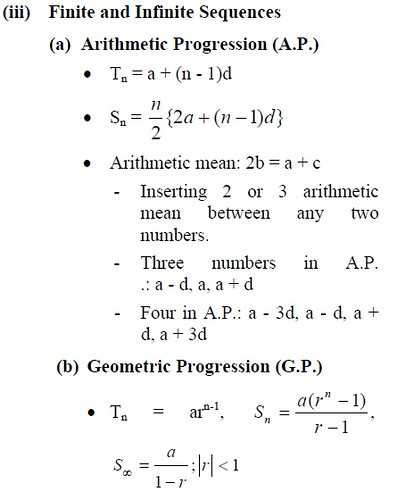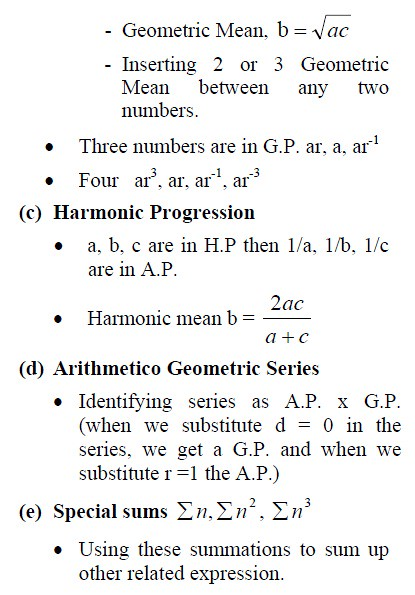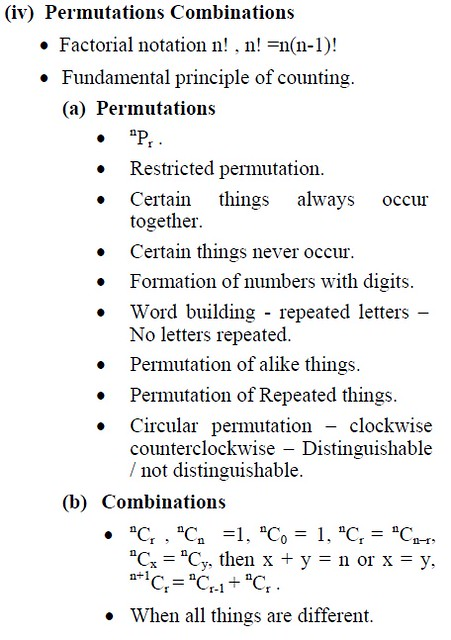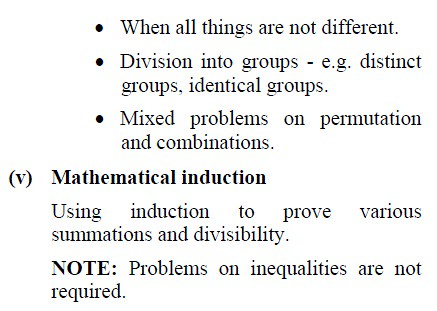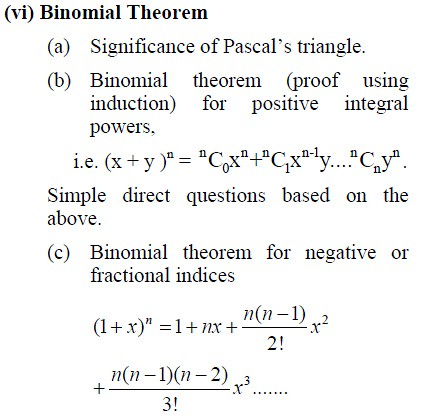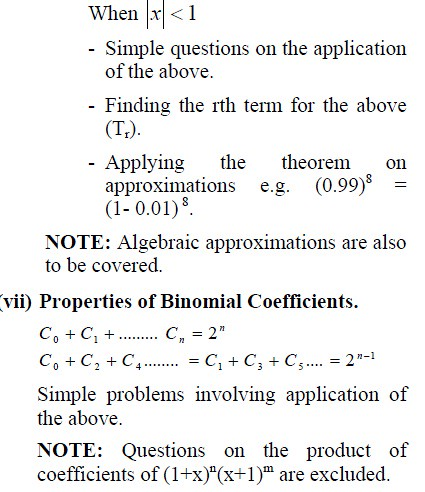3. Trigonometry

(i) Angles and Arc lengths

• Angles: Convention of sign of angles.
• Magnitude of an angle: Measures of Angles; Circular measure.
• The relation S = rθ where θ is in radians. Relation between radians and degree.
• Definition of trigonometric functions with the help of unit circle.
• Truth of the identity sin2 x + cos2 x  = 1.

NOTE: Questions on the area of a sector of a circle are required to be covered.

(ii) Trigonometric Functions

• Relationship between trigonometric functions.
• Proving simple identities.
• Signs of trigonometric functions.
• Domain and range of the trigonometric functions.
• Trigonometric functions of all angles.
• Periods of trigonometric functions.
• Graphs of simple trigonometric functions (only sketches).

NOTE: Graphs of sin x, cos x, tan x, sec x, cosec x and cot x are to be included.

(iii) Compound and multiple angles

• Addition and subtraction formula: sin(AB); cos(AB); Sin(A±B); Cos(A±B);  tan(A±B); tan(A + B + C) etc., Double angle, triple angle, half angle and one third angle formula as special cases.
• Sum and differences as products sinC +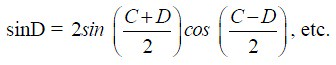• Product to sum or difference i.e. 2sinAcosB = sin(A + B) + sin(A – B) etc.

iv) Trigonometric Equations

• Solution of trigonometric equations (General solution and solution in the specified range).

(a) Equations in which only one function of a single angle is involved e.g. sin 5θ =0
(b) Equations expressible in terms of one trigonometric ratio of the unknown angle.
(c) Equations involving multiple and sub- multiple angles.
(d) Linear equations of the form acosθ + bsinθ = c, where4. Calculus

(i) Basic Concepts of Relations and Functions

(a) Ordered pairs, sets of ordered pairs.
(b) Cartesian Product (Cross) of two sets, cardinal number of a cross product.

Relations as:

• an association between two sets.
• a subset of a Cross Product.

(c) Types of Relations: reflexive, symmetric, transitive and equivalence relation.

(d) Binary Operation.

(e) Domain, Range and Co-domain of a Relation.

(f) Functions:

• As special relations, concept of writing “y is a function of x” as y = f(x).
• Types: one to one/ many to one, into/onto.
• Domain and range of a function.
• Composite function.
• Inverse of a function.
• Classification of functions.
• Sketches of graphs of exponential function, logarithmic function, mod function, step function.

(ii) Differential calculus
(a) Limits

• Notion and meaning of limits.
• Fundamental theorems on limits (statement only).
• Limits of algebraic and trigonometric functions.

NOTE: Indeterminate forms are to be introduced while calculating limits.

(b) Continuity

• Continuity of a function at a point x = a.
• Continuity of a function in an interval.
• Removable discontinuity.

(c) Differentiation

• Meaning and geometrical interpretation of derivative.
• Concept of continuity and differentiability of |x|, [x], etc.
• Derivatives of simple algebraic and trigonometric functions and their formulae.
• Differentiation using first principles.
• Derivatives of sum/difference.
• Derivatives of product of functions.
• Derivatives of quotients of functions.
• Derivatives of composite functions.

NOTE:

1. Derivatives of composite functions using chain rule.
2. All the functions above should be either algebraic or trigonometric in nature.

(d) Application of derivatives

• Equation of Tangent and Normal approximation.
• Rate measure.
• Sign of derivative.
• Monotonocity of a function.

(iii) Integral Calculus

Indefinite integral

• Integration as the inverse of differentiation.
• Anti-derivatives of polynomials and functions (ax +b)n , sinx, cosx, sec2x, cosec2x.
• Integrals of the type sin2x, sin3x, sin4x, cos2x, cos3x, cos4x.

5. Coordinate Geometry

(i) Basic concepts of Points and their coordinates.

(ii) The straight line

• Slope and gradient of a line.
• Angle between two lines.
• Condition of perpendicularity and parallelism.
• Various forms of equation of lines.
• Slope intercept form.
• Two point slope form.
• Intercept form.
• Perpendicular /normal form.
• General equation of a line.
• Distance of a point from a line.
• Distance between parallel lines.
• Equation of lines bisecting the angle between two lines.
• Definition of a locus.
• Methods to find the equation of a locus.

(iii) Circles

Equations of a circle in:

• Standard form.
• Diameter form.
• General form.
• Parametric form.
• Given the equation of a circle, to find the centre and the radius.
• Finding the equation of a circle.

– Given three non collinear points.
– Given other sufficient data that the centre is (h, k) and it lies on a line and two points on the circle are given.

• Tangents:

– Tangent to a circle when the slope of the tangent is given:• Intersection:

– Circle with a line hence to find the length of the chord.

• Finding the equation of a circle through the intersection of two circles i.e. S1 + kS2 = 0.

NOTE: Orthogonal circles are not required to be covered.

6. Statistics

• Measures of central tendency.
• Standard deviation – by direct method, short cut method and step deviation method.
• Combined mean and standard deviation.

NOTE:

1. Combined mean and standard deviation of two groups only are required to be covered.

2. Mean, Median and Mode of grouped and ungrouped data are required to be covered.

SECTION B

7. Vectors

• As directed line segments.
• Magnitude and direction of a vector.
• Types: equal vectors, unit vectors, zero vector.
• Position vector.
• Components of a vector.
• Vectors in two and three dimensions.
•as unit vectors along the x, y and the z axes; expressing a vector in terms of the unit vectors
• Operations: Sum and Difference of vectors; scalar multiplication of a vector.
• Section formula.
• Simple questions based on the above e.g. A line joining the mid point of any two sides of a triangle is parallel to the third side and half of it, concurrency of medians.

8. Co-ordinate geometry in 3-Dimensions

• As an extension of 2-D.
• Distance formula.
• Section and midpoint formula.
• Equation of x-axis, y-axis, z axis and lines parallel to them.
• Equation of xy – plane, yz – plane, zx – plane.
• Direction cosines, direction ratios.
• Angle between two lines in terms of direction cosines /direction ratios.
• Condition for lines to be perpendicular/ parallel.

NOTE: Understanding of dot product of vectors is required.

SECTION C

9. Statistics

• Median – direct and by using the formula.
• Quartiles- direct and by using the formula.
• Deciles- direct and by using the formula.
• Percentiles – direct and by using the formula.
• Mode – graphically, direct method and by using the formula.
• Estimation of median/quartiles from Ogives.

NOTE: The following are also required to be covered:

• The Median, Quartiles, Deciles and Percentiles of grouped and ungrouped data;
• Mode – grouped and ungrouped data; estimation of mode by using graphical method. (Bimodal data not included).

10. Average Due Date

• Zero date.
• Equated periods.

There will be one paper of three hours duration of 100 marks. The syllabus is divided into three sections A, B and C. Section A is compulsory for all candidates. Candidates will have a choice of attempting questions from either Section B or Section C.

Section A (80 marks) will consist of 9 questions. Candidates will be required to answer Question 1(compulsory) and five out of the rest of the eight questions.

Section B/C (20 marks) Candidates will be required to answer two questions out of three from either Section B or Section C.

SECTION A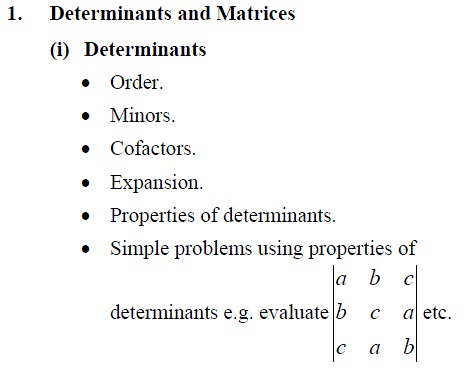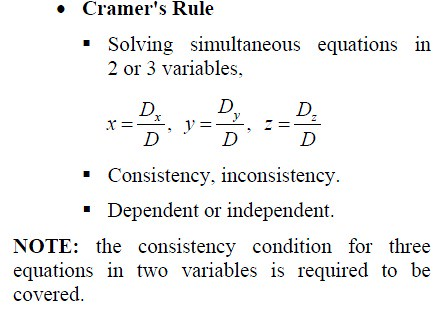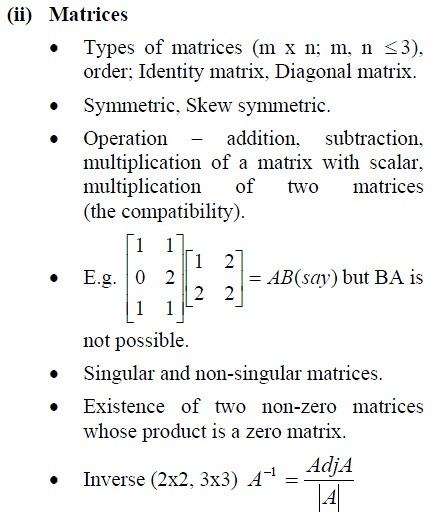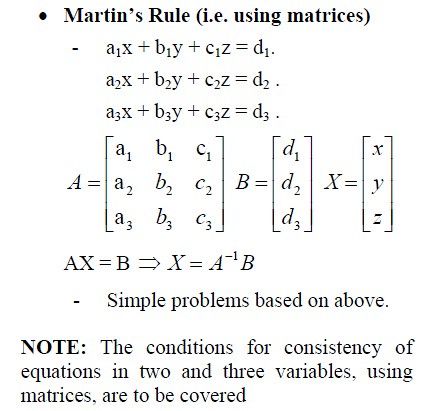2. Boolean Algebra

Boolean algebra as an algebraic structure, principle of duality, Boolean function. Switching circuits, application of Boolean algebra to switching circuits.

3. Conics

• As a section of a cone.
• Definition of Foci, Directrix, Latus Rectum.
• PS = ePL where P is a point on the conics, S is the focus, PL is the perpendicular distance of the point from the directrix.• Rough sketch of the above.
• The latus rectum; quadrants they lie in; coordinates of focus and vertex; and equations of directrix and the axis.
• Finding equation of Parabola when Foci and directrix are given.
• Simple and direct questions based on the above.

(ii) Ellipse

•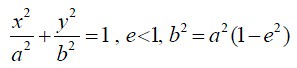• Cases when a > b and a < b.
• Rough sketch of the above.
• Major axis, minor axis; latus rectum; coordinates of vertices, focus and centre; and equations of directrices and the axes.
• Finding equation of ellipse when focus and directrix are given.
• Simple and direct questions based on the above.
• Focal property i.e. SP + SP′ = 2a.

(iii) Hyperbola

Condition that y = mx + c is a tangent to the conics.5. Calculus

(i) Differential Calculus

• Revision of topics done in Class XI – mainly the differentiation of product of two functions, quotient rule, etc.
• Derivatives of trigonometric functions.
• Derivatives of exponential functions.
• Derivatives of logarithmic functions.
• Derivatives of inverse trigonometric functions – differentiation by means of substitution.
• Derivatives of implicit functions and chain rule for composite functions.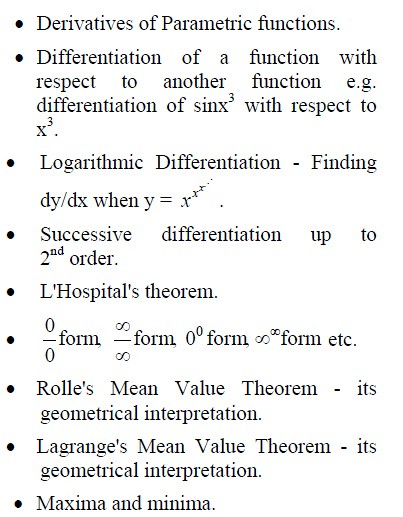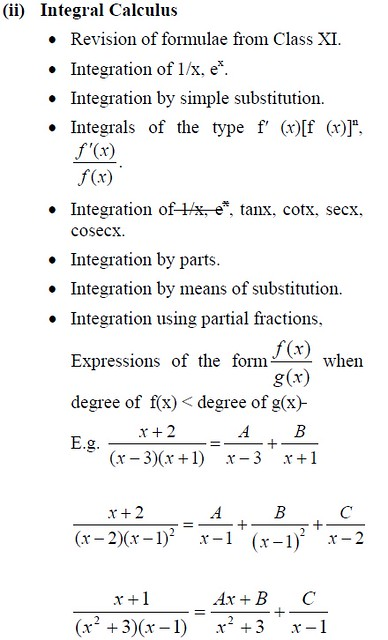Application of definite integrals – area bounded by curves, lines and coordinate axes is required to be covered.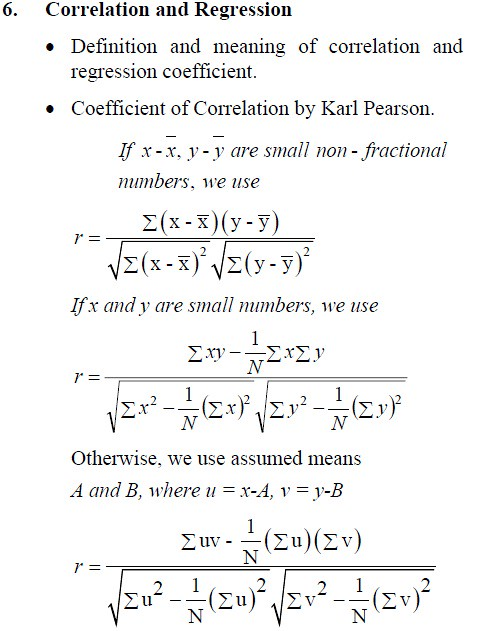7. Probability

• Random experiments and their outcomes.
• Events: sure events, impossible events, mutually exclusive events, independent events and dependent events.
• Definition of probability of an event.
• Laws of probability: addition and multiplication laws, conditional probability (excluding Baye’s theorem).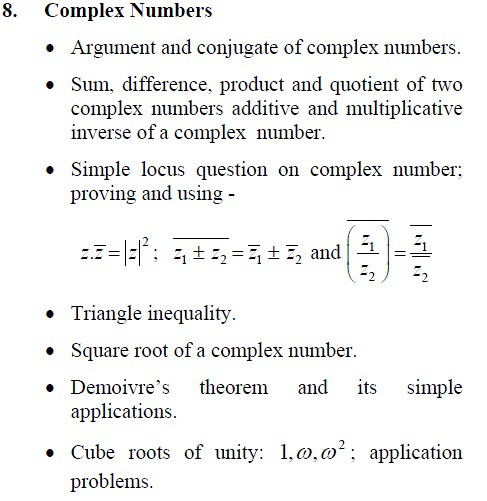9. Differential Equations

• Differential equations, order and degree.
• Solution of differential equations.
• Variable separable.
• Homogeneous equations and equations reducible to homogeneous form.
• Linear form dy/dx=Q where P and Q are functions of x only. Similarly for dx/dy.

NOTE: Equations reducible to variable separable type are included. The second order differential equations are excluded.

SECTION B

10. Vectors

• Scalar (dot) product of vectors.
• Cross product – its properties – area of a triangle, collinear vectors.
• Scalar triple product – volume of a parallelopiped, co-planarity.

Proof of Formulae (Using Vectors)

• Sine rule.
• Cosine rule
• Projection formula
• Area of a Δ = ½absinC

NOTE: Simple geometric applications of the above are required to be covered.

11. Co-ordinate geometry in 3-Dimensions
(i) Lines

• Cartesian and vector equations of a line through one and two points.
• Coplanar and skew lines.
• Conditions for intersection of two lines.
• Shortest distance between two lines.

NOTE: Symmetric and non-symmetric forms of lines are required to be covered.

(ii) Planes

• Cartesian and vector equation of a plane.
• Direction ratios of the normal to the plane.
• One point form.
• Normal form.
• Intercept form.
• Distance of a point from a plane.
• Angle between two planes, a line and a plane.
• Equation of a plane through the intersection of two planes i.e. –

P1 + kP2 = 0.

Simple questions based on the above.

12. Probability

Baye’s theorem; theoretical probability distribution, probability distribution function; binomial distribution – its mean and variance.

NOTE: Theoretical probability distribution is to be limited to binomial distribution only.

SECTION C

13. Discount

True discount; banker’s discount; discounted value; present value; cash discount, bill of exchange.

NOTE: Banker’s gain is required to be covered.

14. Annuities

Meaning, formulae for present value and amount; deferred annuity, applied problems on loans, sinking funds, scholarships.

NOTE: Annuity due is required to be covered.

15. Linear Programming

Introduction, definition of related terminology such as constraints, objective function, optimization, isoprofit, isocost lines; advantages of linear programming; limitations of linear programming; application areas of linear programming; different types of linear programming (L.P.), problems, mathematical formulation of L.P problems, graphical method of solution for problems in two variables, feasible and infeasible regions, feasible and infeasible solutions, optimum feasible solution.
16. Application of derivatives in Commerce and Economics in the following

Cost function, average cost, marginal cost, revenue function and break even point.

17. Index numbers and moving averages

• Price index or price relative.
• Simple aggregate method.
• Weighted aggregate method.
• Simple average of price relatives.
• Weighted average of price relatives (cost of living index, consumer price index).

NOTE: Under moving averages the following are required to be covered:

• Meaning and purpose of the moving averages.
• Calculation of moving averages with the given periodicity and plotting them on a graph.
• If the period is even, then the centered moving average is to be found out and plotted.